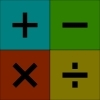You may also likeWorms

Place this "worm" on the 100 square and find the total of the four squares it covers. Keeping its head in the same place, what other totals can you make?Which Scripts?

There are six numbers written in five different scripts. Can you sort out which is which?Highest and Lowest

Put operations signs between the numbers 3 4 5 6 to make the highest possible number and lowest possible number.

One to Fifteen

Age 7 to 11 Challenge Level:

Which circles lie on the fewest straight lines? How might this help?
If you try putting the number $1$ on one circle, where could you put the $2$? Now, where could the $3$ go?
You could use the interactivity to try out your ideas.
You could print off this picture of the circles and use numbered counters to solve the problem, or use a pencil to write numbers in the circles.